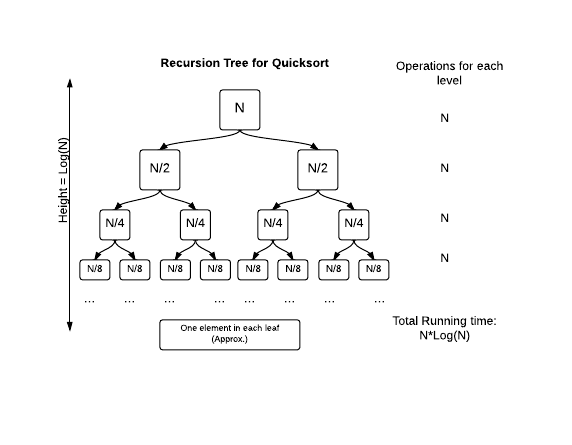# Quick Sort Running Time

The running time of Quicksort will depend on how balanced the partitions are. If you are unlucky enough to always select the greatest or smallest element as the pivot, then each partition will only separate one element at a time, so the running time will be similar to InsertionSort.

However, Quicksort will usually pick a pivot that is mid-range, and it will partition the array into two parts. Let's assume Partition is lucky and it always picks the median element as the pivot. What will be the running time in such a case?

Running Time of Recursive Methods
Quicksort is a recursive method so we will need to use a technique to calculate the total running time of all the method calls. We can use a version of the "Recursion Tree Method" to estimate the running time for a given array of N elements.### Challenge

Compare the number of shifts Insertion Sort and Quicksort take, and output the difference.